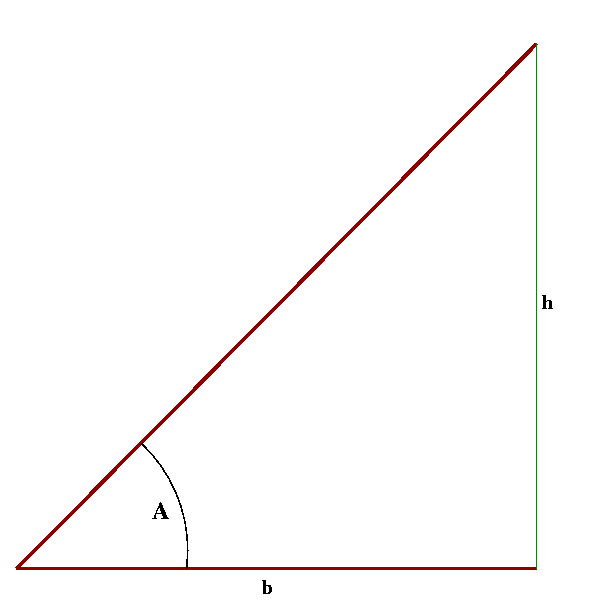The Slope of an Angle

Consider an angle A formed by a horizontal line and a slanted line as in the illustration below.  Such an angle can be specified by just giving its slope.  In the illustration, the slope of angle A is the ratio h/b.  In geometry, this quantity is also referred to as the tangent of the angle A and denoted by Tan(A).  The angle A can also be specified by Cot(A)=b/h, which is called the cotangent of the angle A and is just the inverse of the slope.Here are the slopes of some specific angles:

 15o 0.26795... 30o 0.57735... 45o 1.00000... 60o 1.73205... 75o 3.7321...

Actually, Tan(30o)=1/\/3 ,  Tan(45o)=1, and Tan(60o)=\/3.

Angles associated with a Pyramid

In mathematics, the term pyramid refers to a rather general type of geometric solid.  One can find the definition here.  But the pyramids that one finds in different parts of the world generally have a rectangular base with the apex directly above the center of the base.  Most of the pyramids in Egypt actually have a square base and are therefore quite symmetrical. The four faces are isosceles triangles and are congruent to each other.  The shape of such a pyramid is determined by the angle that each face makes with the base, i.e. the angle formed by the horizontal line joining the midpoint of the base of a face to the center of the pyramid and the slanted line joining that midpoint to the apex of the pyramid.    That angle could be any angle between 0o and 90o. If that angle is close to 90o, then the pyramid would be very tall and would seem like a simple, pointed obelisk.  If that angle is close to 0o, then the pyramid would be almost flat and one would  probably be unhappy calling it a pyramid.  But the shape is also determined by various other quantities.  For example, the angle that each edge of the pyramid makes with the base would be sufficient.  (That angle is formed by the horizontal line joining a corner of the pyramid to the center and the edge joining that corner to the apex.)   Either of these angles is determined by its slope, or the inverse of its slope.  In modern Western Mathematics,  it would be customary to specify either angle by its slope, but the discussions of pyramids that one finds in the Egyptian Mathematical Papyri suggest that the Egyptian architect would have specified an angle by the inverse of its slope. For the slant-angle of the faces of a pyramid, the inverse-slope is referred to as the "seked" of the pyramid in the Rhind papyrus.